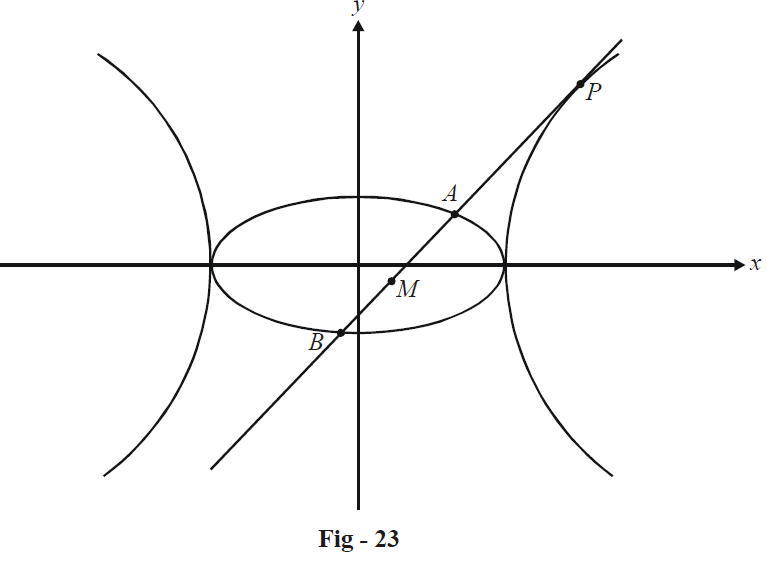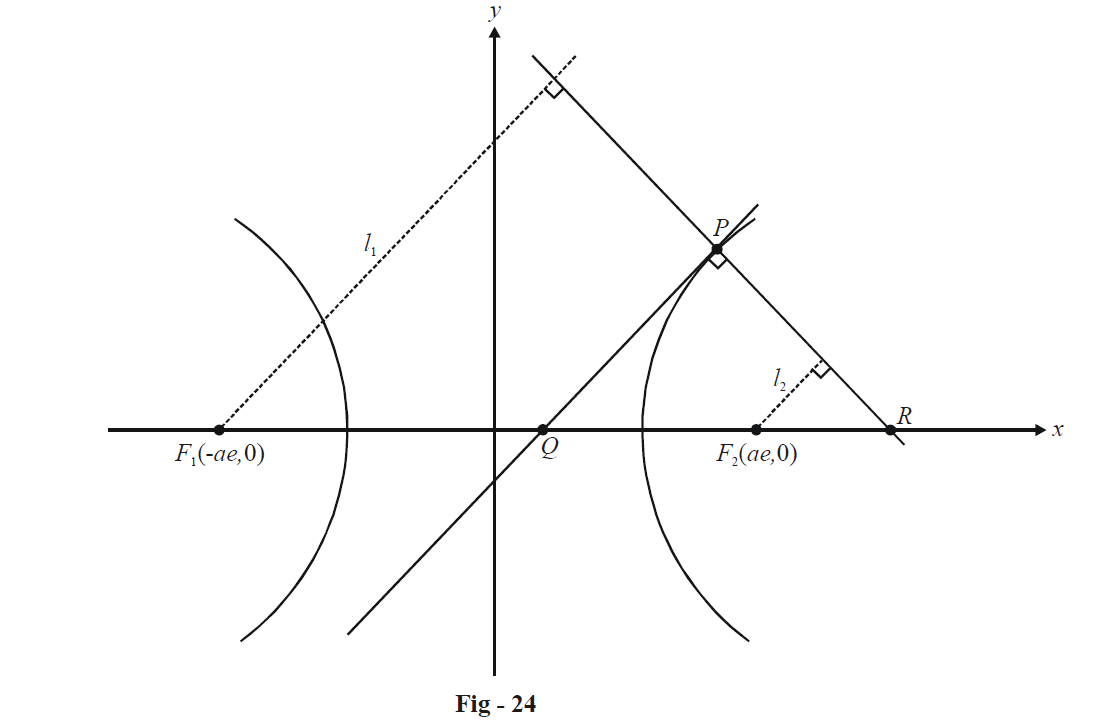# Hyperbolas Set 1

Go back to  'SOLVED EXAMPLES'

Example - 1

A tangent to the hyperbola  \begin{align}\frac{{{x^2}}}{{{a^2}}} - \frac{{{y^2}}}{{{b^2}}} = 1\end{align}  cuts the ellipse  \begin{align}\frac{{{x^2}}}{{{a^2}}} + \frac{{{y^2}}}{{{b^2}}} = 1\end{align}  in points A and B, as shown. Find the locus of the mid-point of AB.Solution: We can assume $$M(h,\,k)$$  to be mid-point of the chord AB. Using the equation for the chord bisected at a given point in an ellipse, the equation of AB is

\begin{align}&\qquad\quad T(h,\,k) = S(h,\,k) \\\\ &\Rightarrow\quad \frac{{hx}}{{{a^2}}} + \frac{{ky}}{{{b^2}}} = \frac{{{h^2}}}{{{a^2}}} + \frac{{{k^2}}}{{{b^2}}} \\\\ &\Rightarrow\quad y = \left( { - \frac{{{b^2}h}}{{{a^2}k}}} \right)x + \frac{{{b^2}}}{k}\left( {\frac{{{h^2}}}{{{a^2}}} + \frac{{{k^2}}}{{{b^2}}}} \right) \\\\ \end{align}

This is a tangent to the given hyperbola if the condition for tangency   $$({c^2} = {a^2}{m^2} - {b^2})$$   is satisfied :

\begin{align}&\qquad\;\;\;\frac{{{b^4}}}{{{k^2}}}{\left( {\frac{{{h^2}}}{{{a^2}}} + \frac{{{k^2}}}{{{b^2}}}} \right)^2} = {a^2}{\left( { - \frac{{{b^2}h}}{{{a^2}k}}} \right)^2} - {b^2} \\ &\Rightarrow\quad {\left( {\frac{{{h^2}}}{{{a^2}}} + \frac{{{k^2}}}{{{b^2}}}} \right)^2} = \frac{{{h^2}}}{{{a^2}}} - \frac{{{k^2}}}{{{b^2}}} \\ \end{align}

Thus, the locus of M is

${\left( {\frac{{{x^2}}}{{{a^2}}} + \frac{{{y^2}}}{{{b^2}}}} \right)^2} = \frac{{{x^2}}}{{{a^2}}} - \frac{{{y^2}}}{{{b^2}}}$

Example - 2

A tangent drawn to the hyperbola   \begin{align}\frac{{{x^2}}}{{{a^2}}} - \frac{{{y^2}}}{{{b^2}}} = 1\end{align}   at the point P intersects its transverse axis in Q. The lengths of the perpendiculars drawn from the two foci  $${F_1}$$ and  $${F_2}$$ upon the normal at P are $${l_1}$$ and  $${l_2}$$ respectively. Prove that PQ is the harmonic mean between $${l_1}$$ and  $${l_2}$$

Solution:Let P be the point   $$(a\sec \theta ,\,b\tan \theta ).$$  The equation of the tangent at P is

$\frac{x}{a}\sec \theta - \frac{y}{b}\tan \theta = 1$

To obtain Q , we put  $$y = 0$$  in this equation ; thus,

$Q \equiv (a\cos \theta ,\,0)$

The normal at P has the equation

$ax\cos \theta + by\cot \theta = {a^2} + {b^2}$

Thus, the point R is

$R \equiv \left( {\frac{{{a^2} + {b^2}}}{a}\sec \theta ,\,0} \right)$

From the figure, observe that

\begin{align}&\qquad\qquad\frac{{{l_2}}}{{R{F_2}}} = \frac{{PQ}}{{RQ}} = \frac{{{l_1}}}{{R{F_1}}} = \lambda \\\\ &\quad\quad\;\Rightarrow\quad {l_1} = \lambda (R{F_1}) = \lambda \left( {\frac{{{a^2} + {b^2}}}{a}\sec \theta + ae} \right) \\\\ & \quad\;\Rightarrow\quad {l_2} = \lambda (R{F_2}) = \lambda \left( {\frac{{{a^2} + {b^2}}}{a}\sec \theta - ae} \right) \\\\ & \quad\;\Rightarrow\quad {l_1}{l_2} = {\lambda ^2}\left( {\frac{{{{\left( {{a^2} + {b^2}} \right)}^2}}}{{{a^2}}}{{\sec }^2}\theta - {a^2}{e^2}} \right) \\\\ & \qquad\qquad\quad= {\lambda ^2}\left( {\frac{{{{\left( {{a^2} + {b^2}} \right)}^2}}}{{{a^2}}}{{\sec }^2}\theta - \left( {{a^2} + {b^2}} \right)} \right) \\\\ &\qquad\qquad\quad= \frac{{{\lambda ^2}({a^2} + {b^2})}}{{{a^2}}}\left( {{a^2}{{\tan }^2}\theta + {b^2}{{\sec }^2}\theta } \right) \\\\ & \qquad\qquad\quad= {\lambda ^2}{e^2}({a^2}{\tan ^2}\theta + {b^2}{\sec ^2}\theta ) \\\\&\text {and}\quad{l_1} + {l_2} = \frac{{2\lambda \sec \theta }}{a}({a^2} + {b^2}) = 2\lambda a{e^2}\sec \theta \end{align}

Thus,

$\frac{{2{l_1}{l_2}}}{{{l_1} + {l_2}}} = \frac{{\lambda ({a^2}{{\tan }^2}\theta + {b^2}{{\sec }^2}\theta )}}{{a\sec \theta }}\,\,\,\,\,\,\,\,\,\,\,\,\,\,\,\,\,\,\,\,\,\,\,\,\,\,\,\,\,\,\,\,\,\,\,\,\,\,\,\,\, \ldots \left( 1 \right)$

Also,

\begin{align}&PQ = \lambda (RQ) \\\\ \,\,\,\,\,\,\,\, &\;\;\;\;\;= \lambda \left( {\frac{{{a^2} + {b^2}}}{a}\sec \theta - a\cos \theta } \right) \\\\ \,\,\,\,\,\,\,\, &\;\;\;\;\;= \lambda \left( {\frac{{({a^2} + {b^2})\sec \theta - {a^2}\cos \theta }}{a}} \right) \\\\ \,\,\,\,\,\,\,\, &\;\;\;\;\;= \lambda \left( {\frac{{{a^2}{{\tan }^2}\theta + {b^2}{{\sec }^2}\theta }}{{a\sec \theta }}} \right)\,\,\,\,\,\,\,\,\,\,\,\,\,\,\,\,\,\,\,\,\,\,\,\,\,\,\,\,\,\,\,\,\,\,\,\,\,\,\, \ldots \left( 2 \right) \\ \end{align}

From (1) and (2), we have

$PQ = \frac{{2{l_1}{l_2}}}{{{l_1} + {l_2}}}$

which means that PQ is the H.M between  $${l_1}$$ and  $${l_1}$$

Learn from the best math teachers and top your exams

• Live one on one classroom and doubt clearing
• Practice worksheets in and after class for conceptual clarity
• Personalized curriculum to keep up with school